Acronym rad (old: rhode) Name rhombic dodecahedron,Voronoi cell of face-centered cubic (fcc) lattice,terminally chamfered cube,terminally chamfered octahedron,surtegmated cube,surtegmated octahedron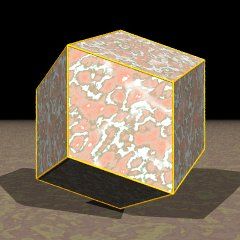© © Inradius sqrt(2/3) = 0.816497 Vertex figure [r4], [R3] Coordinates (1, 1, 1)/sqrt(3)     & all permutations, all changes of sign (3-fold vertices, inscribed sqrt(4/3)-cube) (2, 0, 0)/sqrt(3)     & all permutations, all changes of sign (4-fold vertices, inscribed sqrt(8/3)-oct) General of army (is itself convex) Colonel of regiment (is itself locally convex) Dual co Dihedral angles (at margins) between {(r,R)2} and {(r,R)2}:   120° Confer chamfered cube   chamfered oct Externallinks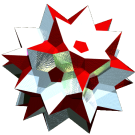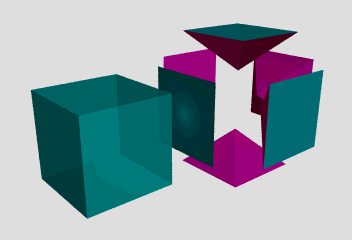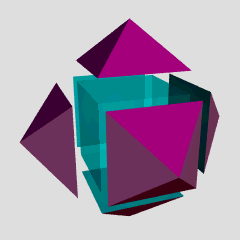©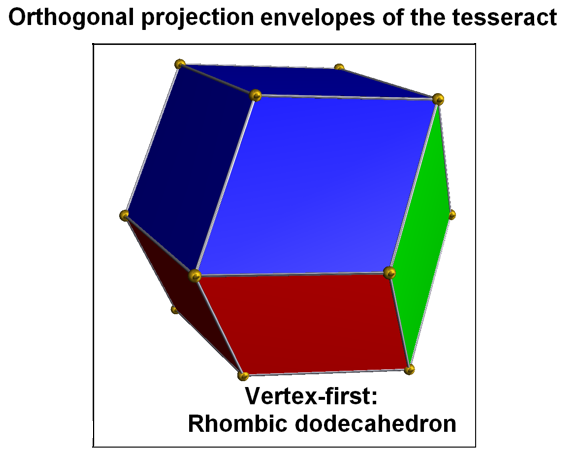©

The rhombs {(r,R)2} have vertex angles r = arccos(1/3) = 70.528779° resp. R = arccos(-1/3) = 109.471221°. Esp. rr : RR = sqrt(2). – Both, the actual shape of the rhombs and the dihedral angle given above, can easily be obtained from the Voronoi complex of the fcc honeycomb, radh, and its relation to the vertex-inscribed primitive cubic honeycomb chon, cf. also the right first 2 pictures.

The last right picture displays that the vertex-first projection of the tes happens to be nothing but rad. In fact, there are exactly two ways of dissecting rad into four congruent rhombohedra, giving a total of eight possible rhombohedra, each a projected cube. The remaining 2 vertices of tes, which are not visible at the surface of rad, thereby get both projected to its body-center. These two rhombohedral dissections then just represent nothing but the front and back cover of the projection pre-image, i.e. of tes. – Thus, re-refering to the previous paragraph, radh happens to be nothing but a single sheet of cover from a likewise projection of test.

All a = rr and b = RR edges, as provided in the below description, only qualify as pseudo edges wrt. the full polyhedron. The true edge size used here is rR = x = 1.

Incidence matrix according to Dynkin symbol

o3m4o =
ao3oo4ob&#zx   → height = 0
a = rr = sqrt(8/3) = 1.632993
b = RR = 2/sqrt(3) = 1.154701

o.3o.4o.     | 6 * |  4 |  4  [r4]
.o3.o4.o     | * 8 |  3 |  3  [R3]
-------------+-----+----+---
oo3oo4oo&#x  | 1 1 | 24 |  2
-------------+-----+----+---
ao .. ob&#zx | 2 2 |  4 | 12  {(r,R)2}

m3o3m =
aoo3oao3ooa&#zx   → height = 0
a = rr = sqrt(8/3) = 1.632993

o..3o..3o..     | 4 * * |  3  0 |  3  [R3]
.o.3.o.3.o.     | * 6 * |  2  2 |  4  [r4]
..o3..o3..o     | * * 4 |  0  3 |  3  [R3]
----------------+-------+-------+---
oo.3oo.3oo.&#x  | 1 1 0 | 12  * |  2
.oo3.oo3.oo&#x  | 0 1 1 |  * 12 |  2
----------------+-------+-------+---
... oao ...&#xt | 1 2 1 |  2  2 | 12  {(r,R)2}